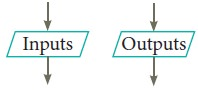Home | | Computer Science 11th std | Notations for Algorithms

# Notations for Algorithms

There are mainly three different notations for representing algorithms.

Notations for Algorithms

We need a notation to represent algorithms. There are mainly three different notations for representing algorithms.

•      A programming language is a notation for expressing algorithms to be executed by computers.

•      Pseudo code is a notation similar to programming languages. Algorithms expressed in pseudo code are not intended to be executed by computers, but for communication among people.

•      Flowchart is a diagrammatic notation for representing algorithms. They give a visual intuition of the flow of control, when the algorithm is executed.

## 1. Programming language

A programming language is a notation for expressing algorithms so that a computer can execute the algorithm. An algorithm expressed in a programming language is called a program. C, C++ and Python are examples of programming languages. Programming language is formal. Programs must obey the grammar of the programming language exactly. Even punctuation symbols must be exact. They do not allow the informal style of natural languages such as English or Tamil. There is a translator which translates the program into instructions executable by the computer. If our program has grammatical errors, this translator will not be able to do the translation.

## 2. Pseudo-code

Pseudo code is a mix of programming-language-like constructs and plain English. This notation is not formal nor exact. It uses the same building blocks as programs, such as variables and control flow. But, it allows the use of natural English for statements and conditions. An algorithm expressed as pseudo code is not for computers to execute directly, but for human readers to understand. Therefore, there is no need to follow the rules of the grammar of a programming language. However, even pseudo code must be rigorous and correct. Pseudo code is the most widely used notation to represent algorithms.

## 3.Flowcharts

Flowchart is a diagrammatic notation for representing algorithms. They show the control flow of algorithms using diagrams in a visual manner. In flowcharts, rectangular boxes represent simple statements, diamond-shaped boxes represent conditions, and arrows describe how the control flows during the execution of the algorithm. A flowchart is a collection of boxes containing statements and conditions which are connected by arrows showing the order in which the boxes are to be executed.

1. A statement is contained in a rectangular box with a single outgoing arrow,which points to the box to be executed next.2. A condition is contained in a diamond-shaped box with two outgoing arrows, labeled true and false. The true arrow points to the box to be executed next if the condition is true, and the false arrow points to the box to be executed next if the condition is false.3. Parallelogram boxes represent inputs given and outputs produced.4. Special boxes marked Start and the End are used to indicate the start and the end of an execution:The flowchart of an algorithm to compute the quotient and remainder after dividing an integer A by another integer B is shown in Figure 7.1, illustrating the different boxes such as input, output, condition, and assignment, and the control flow between the boxes. The algorithm is explained in Example 7.4.(1) Flowcharts are less compact than representation of algorithms in programming language or pseudo code.

(2) They obscure the basic hierarchical structure of the algorithms. (3) Alternative statements and loops are disciplined control flow structures. Flowcharts do not restrict us to disciplined control flow structures.

Study Material, Lecturing Notes, Assignment, Reference, Wiki description explanation, brief detail
11th Computer Science : Chapter 7 : Composition and Decomposition : Notations for Algorithms |